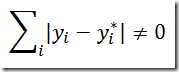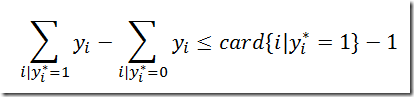## Sunday, October 2, 2011

### Integer cuts

I received a few questions about how to form constraints that exclude a known 0-1 integer solution. Assume our solution we want to exclude is called y* then we can write:This can be linearized as follows:The final result is also sometimes written as the following constraint:Note that y is a binary decision variable.

#### 1 comment:

1.I came across this on StackOverflow. Could you explain why you compare 2*soln - 1 to sum(soln) - 1
WOuldn't sum(soln) give an integer rather than an array?